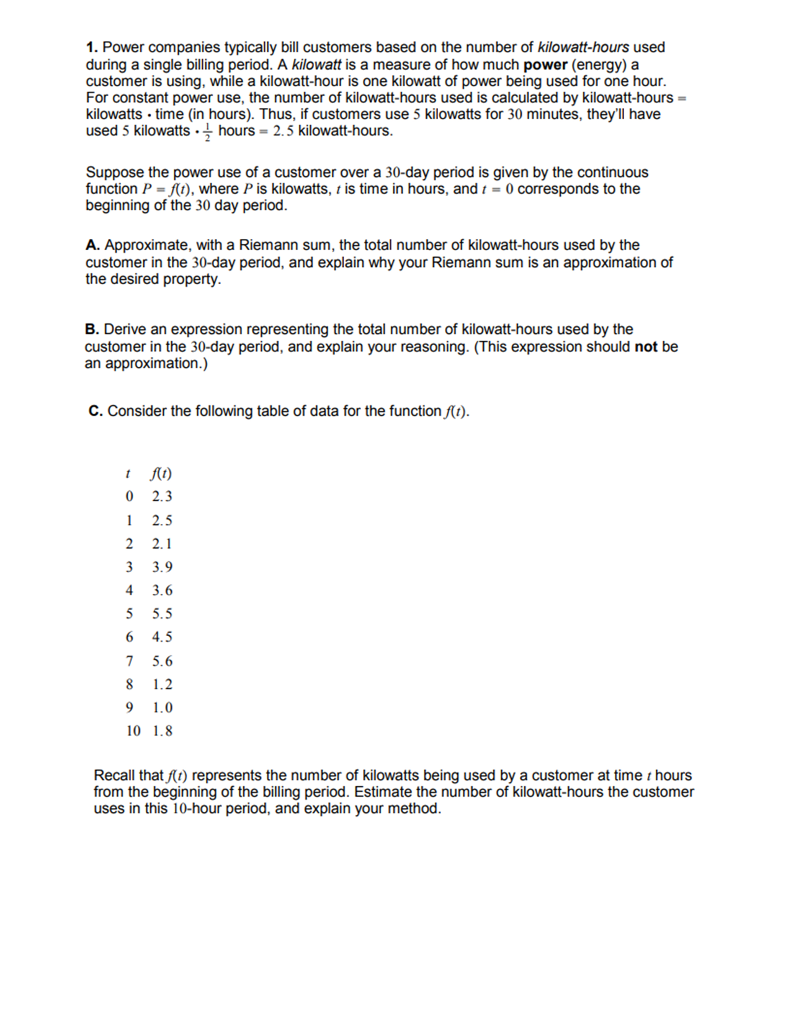# Question 1. Power companies typically bill customers based on the number of kilowatt-hours used during a single billing period. A kilowatt is a measure of how much power (energy) a customer is using, while a kilowatt-hour is one kilowatt of power being used for one hour For constant power use, the number of kilowatt-hours used is calculated by kilowatt-hours 1. Power companies typically bill customers based on the number of kilowatt-hours used during a single billing period. A kilowatt is a measure of how much power (energy) a customer is using, while a kilowatt-hour is one kilowatt of power being used for one hour For constant power use, the number of kilowatt-hours used is calculated by kilowatt-hours kilowatts time (in hours). Thus, if customers use 5 kilowatts for 30 minutes, they'll have used 5 kilowatts hours 2.5 kilowatt-hours Suppose the power use of a customer over a 30-day period is given by the continuous function P =/(1), where P is kilowatts, 1 is time in hours, and 1-0 corresponds to the beginning of the 30 day period A. Approximate, with a Riemann sum, the total number of kilowatt-hours used by the customer in the 30-day period, and explain why your Riemann sum is an approximation of the desired property B. Derive an expression representing the total number of kilowatt-hours used by the customer in the 30-day period, and explain your reasoning. (This expression should not be an approximation.) C. Consider the following table of data for the function/() 0 2.3 1 2.5 2 2.1 3 3.9 4 3.6 5 5.5 6 4.5 7 5.6 9 1.0 10 1.8 Recall that ft) represents the number of kilowatts being used by a customer at time t hours from the beginning of the billing period. Estimate the number of kilowatt-hours the customer uses in this 10-hour period, and explain your methodCLXLKN The Asker · Other MathematicsTranscribed Image Text: 1. Power companies typically bill customers based on the number of kilowatt-hours used during a single billing period. A kilowatt is a measure of how much power (energy) a customer is using, while a kilowatt-hour is one kilowatt of power being used for one hour For constant power use, the number of kilowatt-hours used is calculated by kilowatt-hours kilowatts time (in hours). Thus, if customers use 5 kilowatts for 30 minutes, they'll have used 5 kilowatts hours 2.5 kilowatt-hours Suppose the power use of a customer over a 30-day period is given by the continuous function P =/(1), where P is kilowatts, 1 is time in hours, and 1-0 corresponds to the beginning of the 30 day period A. Approximate, with a Riemann sum, the total number of kilowatt-hours used by the customer in the 30-day period, and explain why your Riemann sum is an approximation of the desired property B. Derive an expression representing the total number of kilowatt-hours used by the customer in the 30-day period, and explain your reasoning. (This expression should not be an approximation.) C. Consider the following table of data for the function/() 0 2.3 1 2.5 2 2.1 3 3.9 4 3.6 5 5.5 6 4.5 7 5.6 9 1.0 10 1.8 Recall that ft) represents the number of kilowatts being used by a customer at time t hours from the beginning of the billing period. Estimate the number of kilowatt-hours the customer uses in this 10-hour period, and explain your method
More
Transcribed Image Text: 1. Power companies typically bill customers based on the number of kilowatt-hours used during a single billing period. A kilowatt is a measure of how much power (energy) a customer is using, while a kilowatt-hour is one kilowatt of power being used for one hour For constant power use, the number of kilowatt-hours used is calculated by kilowatt-hours kilowatts time (in hours). Thus, if customers use 5 kilowatts for 30 minutes, they'll have used 5 kilowatts hours 2.5 kilowatt-hours Suppose the power use of a customer over a 30-day period is given by the continuous function P =/(1), where P is kilowatts, 1 is time in hours, and 1-0 corresponds to the beginning of the 30 day period A. Approximate, with a Riemann sum, the total number of kilowatt-hours used by the customer in the 30-day period, and explain why your Riemann sum is an approximation of the desired property B. Derive an expression representing the total number of kilowatt-hours used by the customer in the 30-day period, and explain your reasoning. (This expression should not be an approximation.) C. Consider the following table of data for the function/() 0 2.3 1 2.5 2 2.1 3 3.9 4 3.6 5 5.5 6 4.5 7 5.6 9 1.0 10 1.8 Recall that ft) represents the number of kilowatts being used by a customer at time t hours from the beginning of the billing period. Estimate the number of kilowatt-hours the customer uses in this 10-hour period, and explain your method